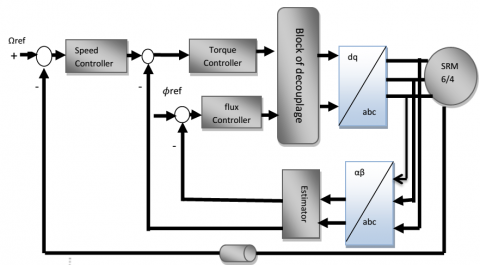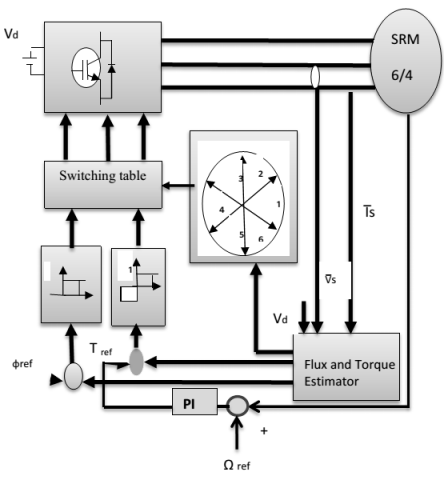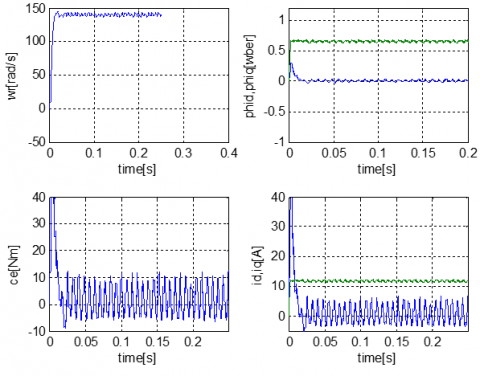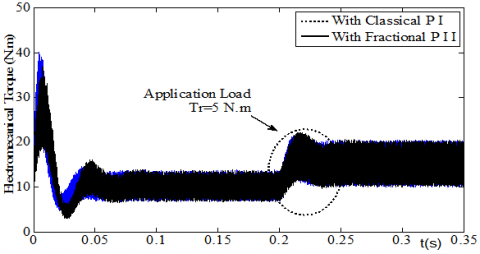# Sizing and Control of a Typical 6/4 Switching Reluctance Motor

Sizing and Control of a Typical 6/4 Switching Reluctance Motor
Electrical engineering department, University Larbi Ben MHIDI, Oum El Bouaghi 04000, Algeria

Corresponding Author Email:
Page:
19-25
|
DOI:
https://doi.org/10.18280/ejee.210104
20 December 2018
|
Revised:
8 Janaury 2019
|
Accepted:
16 Janaury 2019
|
Available online:
15 April 2019
| Citation

OPEN ACCESS

Abstract:

The aim of this study is to present a Switched Reluctance Motor (SRM 6/4) design and control. The use of numerical model for the design of the SRM gives better results compared to the simplified analytical model. The application of the Modified Direct Torque Controller (MDTC) to SRM significantly improves effectiveness of static and dynamic performance compared to field-oriented control and direct torque control, especially in decreasing torque ripple. Finally, the use of a fractional order controller PIaDb allowed the elaboration of a new model on DTC of SRM. The results obtained show a significant performance improvement compared to the classical controller method.

Keywords:

switched reluctance motor 6/4, direct torque control (DTC), field-oriented control (FOC), fractional-order PIaDb controller

1. Introduction

A typical 6/4 Switching Reluctance Motor (SRM 6/4) has a double salient structure where the windings are located at the stator poles and without any kind of magnet at the rotor poles "passive". Three-phase full bridge inverter feds the motor [1-2]. Switched Reluctance Motors are known by high speed operation, simplicity, reduced cost, high degree of independence between phases, robustness, short end-turn and pulsatory torque. In the SRM, motion is produced by the variation of reluctance in the between stator-rotor air gap [1-3].

The characteristics of the SRM such as the flux linkage and the torque, also the variation of magnetic reluctance and the saturation effects are all nonlinear functions of both rotor position and current phase [1-4]. These issues relatively complicate the SRM modelling process. Generally, numerical methods such as Finite Elements Method (FEA), adopted here, are used to handle modelling and optimization problems [5-6].

Regardless the electronic advanced techniques, many studies are devoted to the control of the SRM: Ben Amor  proposed two strategies of a non-linear control, namely: the input-state linearization and the outputs-inputs linearization. The sliding control of the angular position of SRM were studied by Rafael et al. ; whereas, Chouitak , proposed the use of the artificial intelligent strategies, explicitly: neural network, fuzzy-hysteresis. Amri et al.  proposed the application of a neural network as an intelligent software sensor control. Moreover, Pratapgiri et al.  applied the direct torque control (DTC) approach and Birame et al.  added the use of a DTC with 12 sectors. This DTC was based on PWM vector and the fuzzy PI (tuning Rs). The latter offers several advantages such as simple implementation, fast dynamic response, robustness to disturbances, and being capable of mechanical sensor less operation .

Several research efforts have been done to increase the performance of DTC [11-13]. The fractional order control has been effectively applied in various industrial control applications [14-15]. The idea here was in replacing the classical PI controller by fractional-order controller PIaDb.

In this study, a numerical model is presented for sizing a prototype of the SRM type 6/4 by the finite element’s method. To improve the static and dynamic performance of this machine, several techniques are applied and compared for the control, namely: Field-Oriented Control (FOC), Direct Torque Control (DTC), Modified Direct Torque Control (MDTC) and Fractional Order-Direct Torque Control.

2. Optimized Design for 3 Phases 6/4 Poles SRM
3. Model of SRM 6/4

The electrical equations of the SRM in the (d-q) referential frame are:

$\biggr\{\begin{array} Vsd=RsIsd+\frac{dϕsd}{dt}-p\frac{dθ}{dt}ϕsq \\ Vsq=RsIsq+\frac{dϕsd}{dt}-p\frac{dθ}{dt}ϕsq \end{array}$ (2)

The flux equations of the SRM are given by:

$\biggr\{\begin{array} Φsd=Ld Isd \\ Φsq=Lq Isq \end{array}$ (3)

The electromagnetic torque Te is given by:

$T_e=p(Ld-Lq)Isd Isq$  (4)

and the mechanical equation:

$T_e=J\frac{dΩ}{dt}+f.Ω+T_r$ (5)

where Tr is the load torque,  $J$ is the moment of inertia, $Ω$ is the rotor speed and f is the friction factor.

4. Control Technics of SRM 6/4

4.1 Flux Oriented Control (FOC)

The principle of the work of the FOC is obtained when a current-regulated voltage-source inverter drives the motor. The inverter generates a three-phase variable-frequency currents. The speed-loop controller determines the frequencies and amplitudes of the stator currents.

## figure_8.pngFigure 8. Basic flux-oriented control scheme for SRM 6/4

4.2 Direct torque control strategy

The direct torque control method of SRM uses the hysteresis controller of torque, the hysteresis controller of flux and the classical PI controller of speed are shown by Figure 9. The optimal voltage vectors are selected to limit the torque errors and stator flux inside the hysteresis band and then applied to the inverter to reduce the torque and flux errors efficiently and rapidly. The optimal voltage vectors are provided in the form of a switching table [11-12] listed in Table 2.

## figure_9.pngFigure 9. Basic DTC scheme for SRM 6/4 drive

4.2.1 Flux and torque estimation

The stator flux, the position of stator flux and the torque are obtained from the equations (6), (8) and (9), which only use the measured stator voltages and currents.

$\biggr\{\begin{array} ϕsα=\int^t_0(Vsα-Rs Isα)dt \\ϕsβ=\int^t_0(\bar{V}sβ-Rs Isβ)dt \end{array}$ (6)

$\bar{ϕ}s(k+1)≃\bar{ϕ}s(k)+\bar{V}sT$ (7)

$\biggr\{\begin{array} ϕs=\sqrt{ϕsα^2+ϕsβ^2} \\θϕ=arctg\frac{ϕsβ}{ϕsα} \end{array}$ (8)

$Te=\frac{3}{2}p(ϕsαIsα-ϕsβIsβ)$ (9)

To control the electromagnetic torque and the stator flux, the hysteresis controllers are generally used allowing the choice of the suitable sequence. To control the variation of the motor flux, one of the six voltage vectors is selected by the controller from the voltage source inverter [11-12].

## figure_10a.png## figure_10b.pngFigure 10. Choice of the voltage vector

4.2.2 Voltage source inverter

The three- phase voltage of inverter is described as follows:

$\bar{V}_s=\sqrt{\frac{2}{3}}V_d(C_a+C_b^{\frac{2iπ}{3}}+C_c^{\frac{4iπ}{3}})$ (10)

where $(C_a, C_b, C_c)$ are the Boolean switching controls

The hysteresis regulators control the electromagnetic torque and the stator flux. Specifically, a three-level hysteresis comparator controls the torque, whereas a two-level hysteresis comparator controls the stator flux. The Table 2 gives the space voltage vector based on hysteresis regulators and stator flux sector outputs (CTe, Cϕ).

Table 2. Switching table of space voltage vectors

 CTe Cφ S1 S2 S3 S4 S5 S6 1 1 V̅2 V̅3 V̅4 V̅5 V̅6 V̅1 0 1 V̅̅7 V̅0 V̅7 V̅0 V̅7 V̅0 -1 1 V̅6 V̅1 V̅2 V̅3 V̅4 V̅5 1 0 V̅3 V̅4 V̅5 V̅6 V̅1 V̅2 0 0 V̅0 V̅7 V̅0 V̅7 V̅0 V̅7 -1 0 V̅5 V̅6 V̅1 V̅2 V̅3 V̅4

4.3 Modified DTC

To reduce the torque ripple, it’s best to search a new approach of DTC. The idea is to modify the switching table by changing the region of sector of the stator flux vector. The first sector is taken from 0° to 60° instead of -30° to 30°. The main advantage of the modified DTC is to have a constant switching frequency.

Table 3. Switching table for modified DTC

 Cφ CTe S1 S2 S3 S4 S5 S6 1 1 V̅2 V̅3 V̅4 V̅5 V̅6 V̅1 1 0 V̅̅7 V̅0 V̅7 V̅0 V̅7 V̅0 1 -1 V̅1 V̅2 V̅3 V̅4 V̅5 V̅6 0 1 V̅4 V̅5 V̅6 V̅1 V̅2 V̅3 0 0 V̅7 V̅0 V̅7 V̅0 V̅7 V̅0 0 -1 V̅5 V̅6 V̅1 V̅2 V̅3 V̅4

## figure_11.pngFigure 11. The six sectors in the modified DTC

4.4 Fractional DTC of SRM

In order to have a best dynamic behavior, the classical PI controller of figure 9 is replaced by a fractional order controller. Several fractional order controllers are exploited in the literature [14-16]. The Figure 12 displays the block diagram of the proposed fractional order control system based on the bode’s ideal transfer function , which ensures stability and robustness performance of the closed loop over a wide range of operation .

## figure_12.pngFigure 12. Block diagram of the fractional order control system

The process $G_p(s)$ is given by:

$G_p(s)=k_{φm.}G_p1(s)$ (11)

where $k_{φm.}$ is the mechanical torque given in (4) and rewritten as:

$k_{φm}=p(Ld-Lq)$ (12)

and $G_p1(s)$ is the speed transfer function given by:

$G_p1(s)=\frac{1}{J.s+f}=\frac{1}{0.00176.s+0.1}$ (13)

therefore:

$G_p(S)=\frac{kφm}{0.00176.s+0.1}$ (14)

In the given frequency band of interest [10-4 104], the dynamic performance requirement can be satisfied for a phase margin θm=45° and a chosen gain crossover frequency wu=70 rad/s.

Thus, the ideal transfer function can be written as:

$G_m(S)=\frac{70^{1.5}}{s^{1.5}},S=iW$ (15)

5. Simulation Results and Discussion

The Matlab/Simulink programming environment is adopted to study the performance of the Switched Reluctance Motor with FOC, DTC and MDTC. In addition, both of torque and speed responses of SRM6/4 added with DTC are illustrate with PI controller and PIaDb fractional order controller; the motor parameters are listed in Table1.

Figure 13 shows the unload dynamic responses of the speed, flux and torque with flux-oriented control.

Figure 14 shows the performance of SRM with direct torque control in steady and transient states. When the torque load of 1.5Nm is applied to the motor at 0.08s. It is observed that to satisfy the load torque requirements, the electromagnetic torque increases. The trajectory of the stator flux is circular which signify that the errors of flux are limited inside the band hysteresis. We observe also the evolution of the trajectory of stator current in the stator referential (d-q) frame.

Figure 15 shows dynamic performance of SRM with modified direct torque control (MDTC). When the modified DTC is applied, it is clear that the torque and the current have a lower ripple compared to those obtained with classical DTC.

Figure 16 shows the dynamic of speed of SRM6/4 with direct torque control using both of classical PI controller and fractional order PIaDb controller. It is clear that the speed of SRM6/4 with DTC using the fractional order is faster and more stable than DTC using the classical PI.

## figure_13.pngFigure 13. Unload dynamic performance of SRM 6/4 with FOC

## figure_14.pngFigure 14. Dynamic performance of SRM 6/4 with Direct torque control (DTC)

## figure_15.pngFigure 15. Dynamic performance of SRM6/4 with a modified direct torque control (MDTC)

Table 4. PR* in the current and torque for both DTC and MDTC

 Technique PR of Torque PR of Stator Current DTC 17.2 % 13.19 % MDTC 9.6 % 9.46 %
* PR: Percentage Ripple

## figure_16.pngFigure 16. Dynamic of speed response

## figure_17.pngFigure 17. Dynamic of torque response

6. Conclusions

Using a finite elements method, a numerical simulation of electromagnetic model was presented for the design of a typical 6/4 switched reluctance motor. The 2D electromagnetic characteristics are accurately predicted.

It was found that the flux density waveforms generated by the motor were completely non-sinusoidal and its maximum value varies periodically for aligned to unaligned position of the rotor. The simulation results are precisely obtained and they can be exploited directly for the control of typical 6/4 SRM.

The performance of the SRM6/4 as a drive system is analyzed using several techniques of control: Field-Oriented Control (FOC), Direct Torque Control (DTC) and Modified Direct Torque Control (MDTC). The control of magnitude of the flux linkage and the speed change in the stator flux vector command directly the torque. It is noticed that during acceleration the torque is kept in the steady state within the hysteresis band of 10% of rated torque.

Concerning the torque ripples and the waveform of current, it is noticed that the dynamic performances of SRM6/4 are better with modified DTC compared to those found with FOC and classical DTC. The torque and current responses present lower ripple with modified DTC (Table 4).

The simulations results obtained with the fractional order controller show a good dynamic performance in quality of time response, rise time and stability. The robustness and the efficiency of the fractional order DTC design are better compared to DTC with classic PI controller.

Nomenclatures
 V̅s Stator voltage vector, V V̅j voltage space vector number j Sj Sector number j Vsd Component on d axis of the stator voltage Vsq Component on q axis of the stator voltage T The sampling period, µs Vsα Stator voltage component on α axis, V Vsβ Stator voltage component on β axis, V Isd Component on d axis of the current, A Isq Component on q axis of the current, A P Pair-pole number of the SRM machine Lq Quadratic inductance, mH Ld Direct inductance, mH Rs Stator resistance, Ω Te Electromagnetic torque, Nm Js Current density, A/m2 A Potential vector Gp1 Speed transfer function Kφm The mechanical torque, Nm Gm Cj S Bode’s ideal transfer function Boolean switching controls Bode’s variable Greek symbols a Nomination for axis b Nomination for axis ̅ϕ Stator flux vector, wb m Permeability, Ω Rotor speed, rad/s q θϕ Angular position of the rotor, deg. Angular position of flux, deg. Abbreviations DTC Direct Torque Control SRM Switched Reluctance Motor d-q Perpendicular (direct, quadratic) axis FEM Finite Element Method FEA Finite Element Analysis PIaDb Fractional-order   controller MDTC Modified Direct Torque Controller FOC Flux Oriented Control PI PID Proportional Integral controller Proportional-Integral-Derivative controller PR Percentage Ripple
References

 Krishnan R. (2001). Switched Reluctance Motor Drives: Modeling, Simulation, Analysis, Design and Application. CRC Press, ISBN 1420041649. https://doi.org/10.1201/9781420041644

 Vijayraghavan P. (2001). Design of switched reluctance motors and development of a universal controller for switched reluctance and permanent magnet brushless DC motor drives. Ph.D. Thesis. The Faculty of the Virginia Polytechnic Institute, State University, Blacksburg, Virginia.

 Anwar MN, Husain I, Radun A. (2001). A comprehensive design methodology for SRM. IEEE Trans. On Ind. Appl 37(6): 1684-1692. https://doi.org/10.1109/28.968179

 Ilea D. (2011). Conception optimale des moteurs à reluctance variable a commutation electronique pour la traction des véhicules electriques légers. Optimal design of  switched reluctance motors for light electric traction applications. Ph.D. Thesis. Ecole Centrale de Lille et l’Université technique de Cluj-Napoca Roumanie, France.

 Jahan T, Sharifian MBB, Feyzi MR. (2011). Static characteristics of switched reluctance motor 6/4 by finite element analysis. Australian Journal of Basic and Applied Sciences 5(9): 1403-1411.

 Moreau L. (2005). Modelisation,conception et commande de generatrices a reluctance variable basse vitesse. "Modelling,design and command of self excited low speed switched reluctance generator. Ph.D. Thesis. Ecole doctorale sciences et technologies de l’information et des matériaux, Université de Nantes, France.

 Ben AL. (1996). Commande non linéaire d'un moteur à réluctance variable. Non linear control of a variable reluctance motor. Ph.D. Thesis. Département de génie éléctrique et de génie informatique, Université de Montréal, Canada.

 Costa RS, Branco PJ, Pires AJ. (2015). Sliding mode angular position control for an 8/6 switched reluctance machine: Theoretical concept, design and experimental results. Electric Power Systems Research 129: 62-74. https://doi.org/10.1016/j.epsr.2015.07.012

 Chouitak M. (2017). Commande d’un moteur à reluctance variable par l’emploi de l’intelligence artificielle. The control of switched reluctance machine with the use of the artificial intelligence. Ph.D. Thesis. Faculté des sciences et de la technologie, Université d’Oran, Algeria.

 Amri W, Salhi MS, Ben AA. (2014). A new intelligent control strategy of a variable reluctance motor. International Journal of Innovation and Applied Studie (IJIAS) 9(4): 1450-1458.

 Pratapgiri S, Narsimha PPV. (2012). Direct torque control of 4 phase 8/6 switched reluctance motor drive for constant torque load. World Journal of Modelling and Simulation(WJMS) 8(3): 185-193.

 Birame M. (2015). Commande sans capteur de vitesse d’un moteur à reluctance variable par l’utilisation des techniques de l’intelligences artificielle. speed sensorless Direct Torque Control for a switched synchronous machine (RSM) with the use of  the intelligent artificial strategies. Ph.D. Thesis. Faculté des sciences de l’ingénieur, Université de Batna, Algeria.

 Sivaprakasam A, Matiigandan T. (2013). Novel switching table for direct torque controlled permanent magnet synchronous motors to reduce torque ripple. Journal of Power Electronics (JPE) 13(6): 939-954. https://doi.org/10.6113/JPE.2017.17.2.368

 Rinku S, Subhransu P, Gagandeep K. (2012). Design of fractional order PID controller for speed control of DC motor. International Journal of Scientific and Research Publications 2(6): 2250-3153.

 Chunna Z, Xiang Z. (2008). The application of fractional order PID controller to position servomechanism. Proceedings of the 7th World Congress on Intelligent Control and Automation. Chongqing, China, pp. 52-27. https://doi.org/10.1109/WCICA.2008.4593462

 Oustaloup A, Levron F, Nanot FM, Mathieu B. (2000). Frequency band complex noninteger differentiator: characterization and synthesis. IEEE Transactions on Circuits Systems I: Fundam. Theory Applications (IEEE) 47(1): 25-39. https://doi.org/10.1109/81.817385

 Djouambi A, Charef A, Bouktir T. (2005). Fractional order robust control and PIλDμ controllers. WSEAS Transaction on Circuits and Systems 4(8): 850- 857.

 Ramiro S, Barbosa JA, Machado T, Ferreira IM. (2003). A fractional calculus perspective of PID tuning. proceedings of DETC. 03ASME Design Engineering Technical Conferences and Computers and Information in Engineering Conference, Chicago, Illinois, USA.